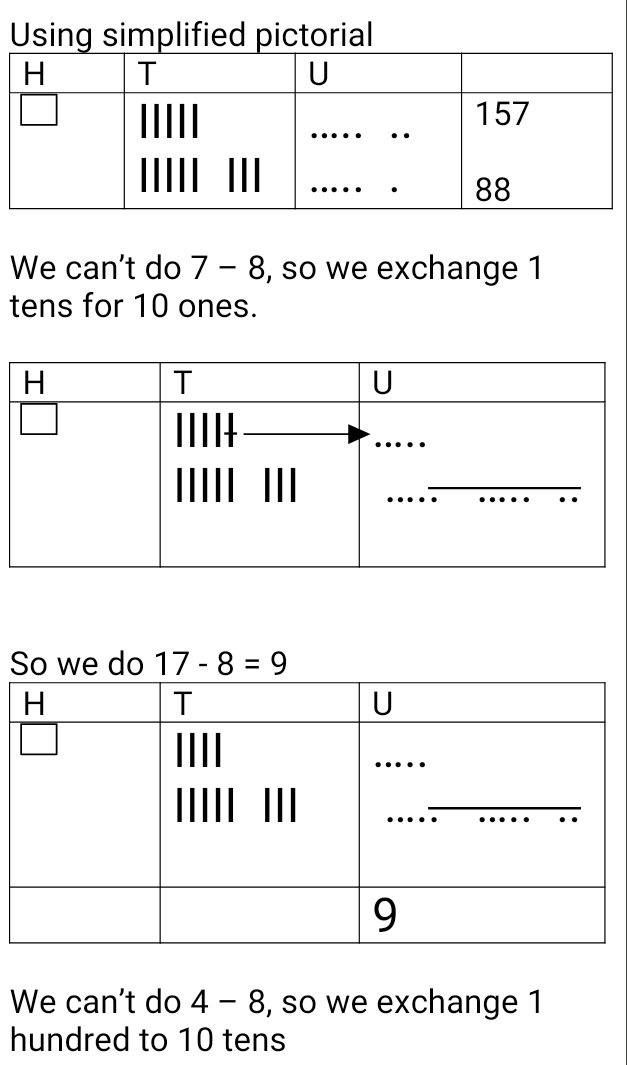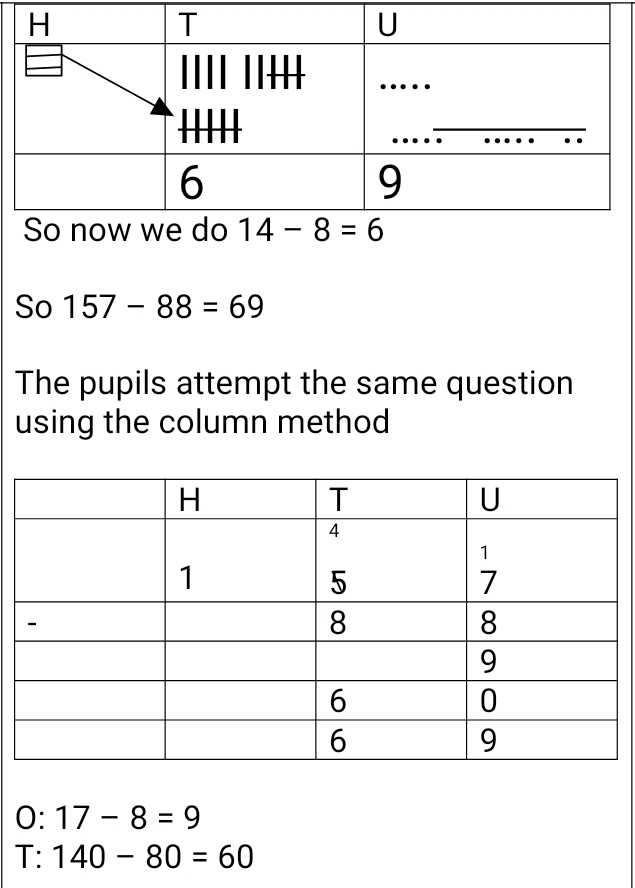# Lesson Notes By Weeks and Term - Primary 3

TERM: 1st Term

WEEK: 7

CLASS: Primary 3

AGE: 8 years

DURATION: 5 periods of 40 minutes each

DATE:

SUBJECT: Mathematics

SPECIFIC OBJECTIVES: At the end of the lesson, the pupils should be able to

1. Solve addition and subtraction problems using various strategies

INSTRUCTIONAL TECHNIQUES: Explanation, question and answer, demonstration, practical, assessments

INSTRUCTIONAL MATERIALS: Base 10 kit, 100 number board, scrap paper, videos from source- https://www.youtube.com/watch?v=_1Z9SQHB5J0

PERIOD 1: Subtraction using the column method

 PRESENTATION TEACHER’S ACTIVITY PUPIL’S ACTIVITY STEP 1MENTAL MATHS The teacher begins the lesson with some mental calculationsCalculate1. 10 -3 =2. 20 – 5 =3. 30 – 6 =4. 40 – 1 =5. 50 – 8 =6. 60 – 2 =7. 70 – 4 =8. 80 – 7 =9. 90 – 9 =10. 100 – 10 = Pupils respond and participate STEP 2CONCEPTDEVELOPMENT The teacher writes 105 – 46 = on the board. Learners are asked to solve this using the base ten kits or simplified pictorialsUse the same process to solve102 – 9 =100 – 7 =132 – 128 = The teacher also explains how to check if the solution to a subtraction problem is correct 105 – 46 = 59Check: 59 + 46 = 105 Pupils pay attention and participate STEP 3CLASS-WORK 1. Use column method and simplified pictorials to solve the followinga. 105 – 56 =b. 103 – 49 =c. 106 – 9 =d. 100 – 4 =e. 142 – 138 = Pupils attempt their class work STEP 4HOME-WORK 1. Use column method and simplified pictorials to solve the followinga. 107 – 49 =b. 108 – 9 =c. 121 – 116 = Pupils attempt their class work STEP 5SUMMARY The teacher summarizes reminding the pupils of the steps in carrying out subtraction using the simplified pictorial and the column method and also what to do when there is a zero in the tens or the ones place She marks their class works, makes corrections where necessary and commends them positively

PERIOD 2: Subtraction using various strategies

 PRESENTATION TEACHER’S ACTIVITY PUPIL’S ACTIVITY STEP 1MENTAL MATHS The teacher begins the lesson with some mental calculationsCalculate1. 100 - 70 =2. 100 – 20 =3. 100 – 50 =4. 100 – 60 =5. 100 – 40 =6. 100 – 90 =7. 100 – 30 =8. 100 – 80 =9. 100 – 10 =10. 100 – 100 = Pupils respond and participate STEP 2CONCEPTDEVELOPMENT The teacher writes 157 – 88 = on the board. Learners are asked to solve this using the base ten kits or simplified pictorials A learner is asked to show the solution to the question on the boardUse the same process to solve143 – 89 =186 – 99 =124 – 65 = The teacher also explains how to check if the solution to a subtraction problem is correct 157 – 88 = 69Check: 69 + 88 = 157 Pupils pay attention and participate STEP 3CLASS-WORK 1. Use column method and simplified pictorials to solve the followinga. 136 – 97 =b. 166 – 78 =c. 144 – 99 =d. 192 – 98 =e. 155 – 79 =f. 147 – 69 =g. 165 – 86 = Pupils attempt their class work STEP 4HOME-WORK 1. Use column method and simplified pictorials to solve the followinga. 158 – 79 =b. 111 – 22 =c. 143 – 86 =d. 137 – 18 = Pupils attempt their class work STEP 5SUMMARY The teacher summarizes reminding the pupils of the steps in carrying out subtraction using the simplified pictorial and the column method , what to do when there is a zero in the tens or the ones place and how to check whether the solution is correct She marks their class works, makes corrections where necessary and commends them positively

PERIOD 3: Subtraction

 PRESENTATION TEACHER’S ACTIVITY PUPIL’S ACTIVITY STEP 1ORALASSESSMENTS The teacher asks questions on mental maths with 2-digit numbers treated so far Pupils respond and participate STEP 2DISCUSSION The teacher discusses all the methods used by some learners in the oral assessments(some of the questions are solved on the board by the learners) and addresses any misconceptions that may have risen Pupils pay attention and participate STEP 3WRITTEN ASSESSMENTS 1 Solve the following using simplified pictoriala. 117 - 68 =b. 133 - 94 =c. 171 - 99 =d. 133 - 85 = 2. Solve the following using the column methoda. 103 - 64=b. 178 - 89 =c. 165 - 89 =d. 143 - 86 = Pupils attempt their class work STEP 4SUMMARY The teacher marks the written assessments, corrects were necessary and commends the pupils

PERIOD 4: Addition and Subtraction using column method

PRESENTATION

TEACHER’S ACTIVITY

PUPIL’S ACTIVITY

STEP 1

MENTAL MATHS

The teacher begins the lesson with some mental calculations

Calculate

1. 100 + 45 =

2. 300 + 53 =

3. 500 + 67 =

4. 700 + 82 =

5. 900 + 5 =

6. 245 – 45 =

7. 464 – 64 =

8. 628 – 28 =

9. 853 – 53 =

10. 709 – 9 =

Pupils respond and participate

STEP 2

CONCEPT

DEVELOPMENT

The teacher writes 12 + 35 = _____ on the board and asks the learners to solve the question using column method in their class work book

Solution

 T U 1 2 + 3 5 4 7

The teacher writes 512 + 35 = ______ on the board and asks the learners to solve the question using the column method in their class work book

Solution

 H T U 5 1 2 + 3 5 5 4 7

The teacher writes 38 – 25 = _____ on the board and the learners are to provide the solution in their class work books

Solution

 T U 3 8 - 2 5 1 3

The teacher writes 438 – 25 = _____ on the board and the learners are to provide the solution in their class work books

Solution

 H T U 4 3 8 - 2 5 4 1 3

Learners in pairs are to solve the following

314 + 73 =

576 – 34 =

Pupils pay attention and participate

STEP 3

CLASS-WORK

Solve the following using column method a. 352 + 44=

b. 463 + 24 =

c. 327 + 51=

d. 546 + 23 =

e. 713 + 55=

f. 295 – 31 =

g. 479 – 46 =

h. 589 – 54 =

i. 672 – 62 =

j. 899 - 98

Pupils attempt their class work

STEP 4

HOME-WORK

Solve the following using column method

a. 737 + 51 =

b. 327 + 22 =

c. 376 - 32 =

d. 654 – 42 =

The pupils writes it in their homework book

STEP 5

SUMMARY

The teacher reminding the pupils how simple addition subtraction problems were solved using column method

She marks the class work, make corrections where necessary and commends the pupils positively

PERIOD 5: Weekly Test/consolidations

TEACHER’S ACTIVITY: The teacher revises all the concepts treated from period 1-4 and gives the pupils follow through exercises, quiz and tests . She marks the exercises, makes corrections and commends the pupils positively.

PUPIL’S ACTIVITY: The pupils work on the worksheets and exercises given by the teacher individually

ASSESSMENT

1 Solve the following using the column method:

a 43 + 68 =

b 88 + 74 =

c 146 – 77 =

d 191 – 45 =

f 103 – 8 =

2 Solve the following using the simplified pictorials:

a 52 + 79 =

b 146 - 129 =

3. Solve the following using any method of your choice. Include a check for correct answers in the end

a 542 – 19 =

b 381 – 49 =